# CH 2.pptx

20 de Aug de 2022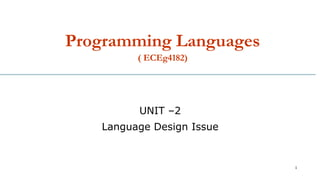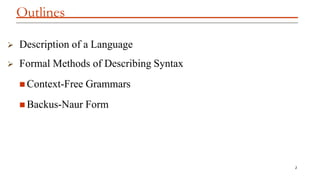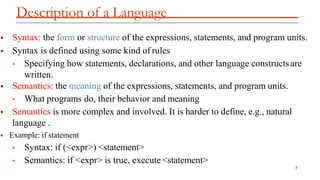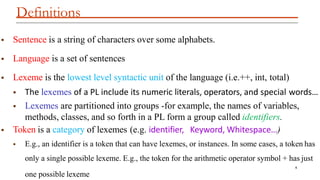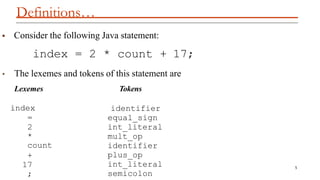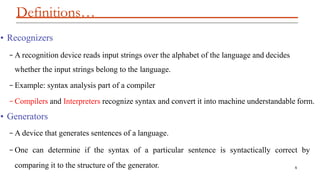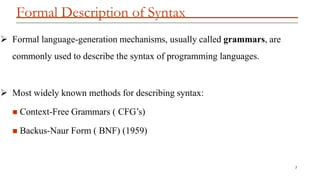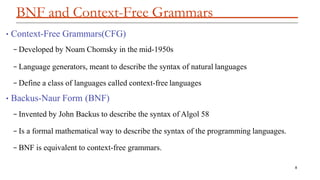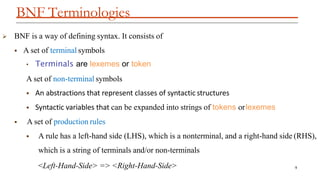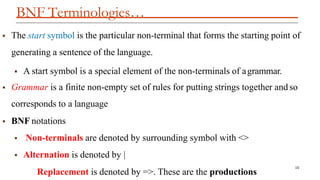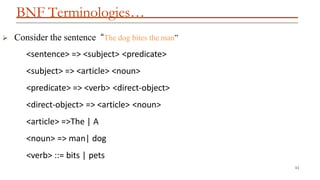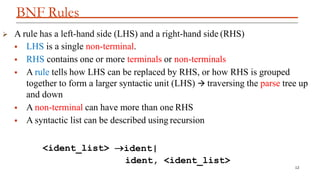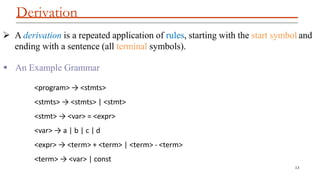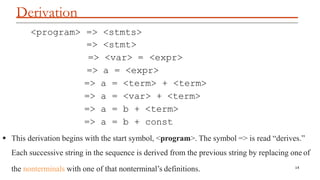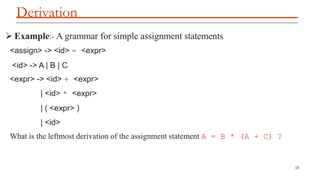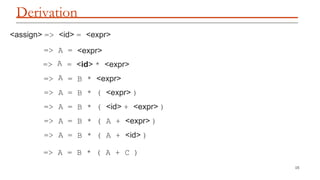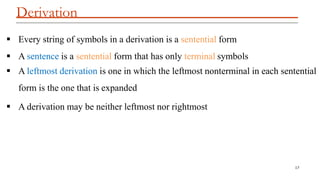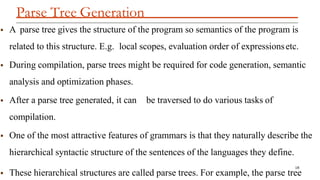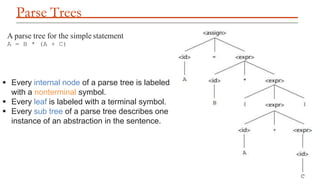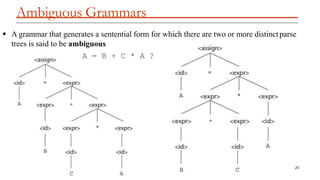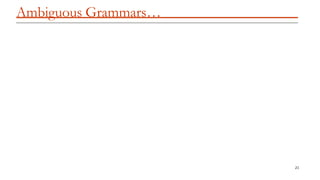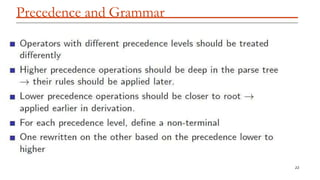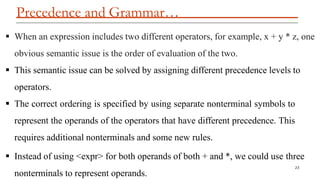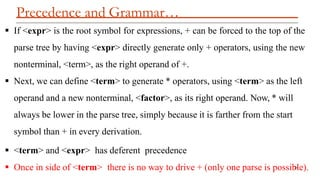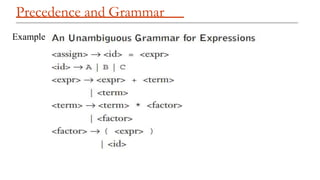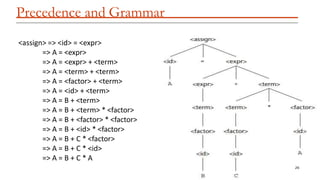1 de 26

### CH 2.pptx

• 1. Programming Languages ( ECEg4182) 1 UNIT –2 Language Design Issue
• 2. Outlines 2  Description of a Language  Formal Methods of Describing Syntax  Context-Free Grammars  Backus-Naur Form
• 3. Description of a Language 3  Syntax: the form or structure of the expressions, statements, and program units.  Syntax is defined using some kind of rules • Specifying how statements, declarations, and other language constructsare written.  Semantics: the meaning of the expressions, statements, and program units. • What programs do, their behavior and meaning  Semantics is more complex and involved. It is harder to define, e.g., natural language .  Example: if statement • Syntax: if (<expr>) <statement> • Semantics: if <expr> is true, execute <statement>
• 4. Definitions 4 one possible lexeme  Sentence is a string of characters over some alphabets.  Language is a set of sentences  Lexeme is the lowest level syntactic unit of the language (i.e.++, int, total)  The lexemes of a PL include its numeric literals, operators, and special words…  Lexemes are partitioned into groups -for example, the names of variables, methods, classes, and so forth in a PL form a group called identifiers.  Token is a category of lexemes (e.g. identifier, Keyword, Whitespace…)  E.g., an identifier is a token that can have lexemes, or instances. In some cases, a token has only a single possible lexeme. E.g., the token for the arithmetic operator symbol + has just
• 5. Definitions… 5  Consider the following Java statement: index = 2 * count + 17; • The lexemes and tokens of this statement are Lexemes Tokens index = 2 identifier equal_sign int_literal mult_op identifier plus_op int_literal semicolon * count + 17 ;
• 6. Definitions… 6 • Recognizers – A recognition device reads input strings over the alphabet of the language and decides whether the input strings belong to the language. – Example: syntax analysis part of a compiler – Compilers and Interpreters recognize syntax and convert it into machine understandable form. • Generators – A device that generates sentences of a language. – One can determine if the syntax of a particular sentence is syntactically correct by comparing it to the structure of the generator.
• 7. Formal Description of Syntax 7  Formal language-generation mechanisms, usually called grammars, are commonly used to describe the syntax of programming languages.  Most widely known methods for describing syntax:  Context-Free Grammars ( CFG’s)  Backus-Naur Form ( BNF) (1959)
• 8. BNF and Context-Free Grammars 8 •Context-Free Grammars(CFG) – Developed by Noam Chomsky in the mid-1950s – Language generators, meant to describe the syntax of natural languages – Define a class of languages called context-free languages •Backus-Naur Form (BNF) – Invented by John Backus to describe the syntax of Algol 58 – Is a formal mathematical way to describe the syntax of the programming languages. – BNF is equivalent to context-free grammars.
• 9. BNF Terminologies 9  BNF is a way of defining syntax. It consists of  A set of terminal symbols • Terminals are lexemes or token A set of non-terminal symbols  An abstractions that represent classes of syntactic structures  Syntactic variables that can be expanded into strings of tokens orlexemes  A set of production rules  A rule has a left-hand side (LHS), which is a nonterminal, and a right-hand side (RHS), which is a string of terminals and/or non-terminals <Left-Hand-Side> => <Right-Hand-Side>
• 10. BNF Terminologies… 10  The start symbol is the particular non-terminal that forms the starting point of generating a sentence of the language.  A start symbol is a special element of the non-terminals of agrammar.  Grammar is a finite non-empty set of rules for putting strings together and so corresponds to a language  BNF notations  Non-terminals are denoted by surrounding symbol with <>  Alternation is denoted by | Replacement is denoted by =>. These are the productions
• 11. BNF Terminologies… 11  Consider the sentence “The dog bites the man” <sentence> => <subject> <predicate> <subject> => <article> <noun> <predicate> => <verb> <direct-object> <direct-object> => <article> <noun> <article> =>The | A <noun> => man| dog <verb> ::= bits | pets
• 12. BNF Rules 12  A rule has a left-hand side (LHS) and a right-hand side (RHS)  LHS is a single non-terminal.  RHS contains one or more terminals or non-terminals  A rule tells how LHS can be replaced by RHS, or how RHS is grouped together to form a larger syntactic unit (LHS)  traversing the parse tree up and down  A non-terminal can have more than one RHS  A syntactic list can be described using recursion <ident_list> ident| ident, <ident_list>
• 13. Derivation 13  A derivation is a repeated application of rules, starting with the start symbol and ending with a sentence (all terminal symbols).  An Example Grammar <program> → <stmts> <stmts> → <stmts> | <stmt> <stmt> → <var> = <expr> <var> → a | b | c | d <expr> → <term> + <term> | <term> - <term> <term> → <var> | const
• 14. Derivation 14 <program> => <stmts> => <stmt> => <var> = <expr> => a = <expr> => a = <term> + <term> => a = <var> + <term> => a = b + <term> => a = b + const  This derivation begins with the start symbol, <program>. The symbol => is read “derives.” Each successive string in the sequence is derived from the previous string by replacing one of the nonterminals with one of that nonterminal’s definitions.
• 15. Derivation 15  Example:- A grammar for simple assignment statements <assign> -> <id> = <expr> <id> -> A | B | C <expr> -> <id> + <expr> | <id> * <expr> | ( <expr> ) | <id> What is the leftmost derivation of the assignment statement A = B * (A + C) ?
• 16. Derivation 15 <assign> => <id> = <expr> => => => A A A = = = <expr> <id> * <expr> B * <expr> => A = B * ( <expr> ) => A = B * ( <id> + <expr> ) => A = B * ( A + <expr> ) => A = B * ( A + <id> ) => A = B * ( A + C )
• 17. Derivation 17  Every string of symbols in a derivation is a sentential form  A sentence is a sentential form that has only terminal symbols  A leftmost derivation is one in which the leftmost nonterminal in each sentential form is the one that is expanded  A derivation may be neither leftmost nor rightmost
• 18. Parse Tree Generation 18  These hierarchical structures are called parse trees. For example, the parse tree  A parse tree gives the structure of the program so semantics of the program is related to this structure. E.g. local scopes, evaluation order of expressionsetc.  During compilation, parse trees might be required for code generation, semantic analysis and optimization phases.  After a parse tree generated, it can be traversed to do various tasks of compilation.  One of the most attractive features of grammars is that they naturally describe the hierarchical syntactic structure of the sentences of the languages they define.
• 19. Parse Trees 19 A parse tree for the simple statement A = B * (A + C)  Every internal node of a parse tree is labeled with a nonterminal symbol.  Every leaf is labeled with a terminal symbol.  Every sub tree of a parse tree describes one instance of an abstraction in the sentence.
• 20. Ambiguous Grammars  A grammar that generates a sentential form for which there are two or more distinctparse trees is said to be ambiguous A = B + C * A ? 20
• 21. Ambiguous Grammars… 21
• 22. Precedence and Grammar 22
• 23. Precedence and Grammar… 23 nonterminals to represent operands.  When an expression includes two different operators, for example, x + y * z, one obvious semantic issue is the order of evaluation of the two.  This semantic issue can be solved by assigning different precedence levels to operators.  The correct ordering is specified by using separate nonterminal symbols to represent the operands of the operators that have different precedence. This requires additional nonterminals and some new rules.  Instead of using <expr> for both operands of both + and *, we could use three
• 24. Precedence and Grammar…  If <expr> is the root symbol for expressions, + can be forced to the top of the parse tree by having <expr> directly generate only + operators, using the new nonterminal, <term>, as the right operand of +.  Next, we can define <term> to generate * operators, using <term> as the left operand and a new nonterminal, <factor>, as its right operand. Now, * will always be lower in the parse tree, simply because it is farther from the start symbol than + in every derivation.  <term> and <expr> has deferent precedence  Once in side of <term> there is no way to drive + (only one parse is possib 24 le).
• 25. Precedence and Grammar Example
• 26. Precedence and Grammar <assign> => <id> = <expr> => A = <expr> => A = <expr> + <term> => A = <term> + <term> => A = <factor> + <term> => A = <id> + <term> => A = B + <term> => A = B + <term> * <factor> => A = B + <factor> * <factor> => A = B + <id> * <factor> => A = B + C * <factor> => A = B + C * <id> => A = B + C * A 26